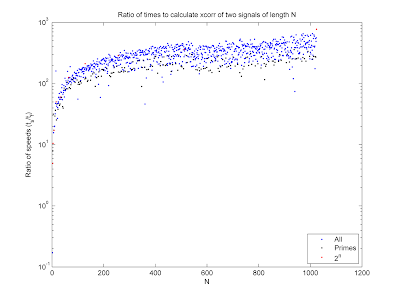## Friday, June 5, 2009

### Fast Correlation

I've uploaded a small package to the Matlab File Exchange to improve the speed of your cross correlations. This package uses the FFT to calculate the circular cross correlation of two periodic signals. Since the FFT calculates the DFT of a N-point signal in O(N log N) calculations (rather than O(N2)) it is much faster than the naïve method, especially for long signals. In Matlab, the fft method is faster than the multiplication method in all but the most trivial cases, as shown in the image, plotting the ratio of the speed of the fast method to that of the naïve method from the definition. The figure also shows that the fft algorithm is typically fastest when N is a power of 2, and slowest when N is prime.